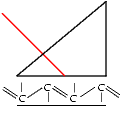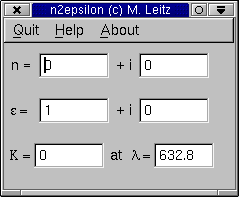# N2EpsilonThis little program -n2epsilon- turns values for the refractive index (n = n´+i*kappa ) into values for the dielectric constant (epsilon = epsilon´+i*epsilon´´ ) and vice versa.
It performs a very simple calculation with the complex values according to:

n = sqrt(epsilon) and epsilon = n^2

In addition it display the absorption constant according to:

K = 2*Pi/lambda * kappa

Where lambda denotes the wavelength of light. Note, that the unit of K is [1/m] !! The program provides two input fields for n and epsilon respectively. After changing one of the four parameters the corrosponding two other parameter will be recalculated.
It is possible to change the wavelength. In this case K is recalculated. The unit of lambda is [nm].